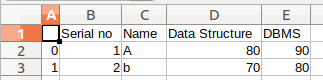# Create a GUI to convert CSV file into excel file using Python

Prerequisites: Python GUI – tkinter, Read csv using pandas

CSV file is a Comma Separated Value file that uses a comma to separate values. It is basically used for exchanging data between different applications. In this, individual rows are separated by a newline. Fields of data in each row are delimited with a comma.

### Modules Needed

• Pandas: Python is a great language for doing data analysis, primarily because of the fantastic ecosystem of data-centric python packages. To install this module type the below command in the terminal.
`pip install pandas`
• pandastable: This library provides a table widget for Tkinter with plotting and data manipulation functionality. To install this module type the below command in the terminal
`pip install pandastable`
• tkintertable: This library is used for adding tables to a Tkinter application. To install this library type the below command in the terminal.
`pip install tkintertable`

Below is the implementation.

Input CSV File:`import` `pandas as pd ` `from` `tkinter ``import` `*` `from` `tkinter ``import` `filedialog ` `from` `tkinter ``import` `messagebox as msg ` `from` `pandastable ``import` `Table ` `from` `tkintertable ``import` `TableCanvas ` `  `  ` `  `class` `csv_to_excel: ` `  `  `    ``def` `__init__(``self``, root): ` `  `  `        ``self``.root ``=` `root ` `        ``self``.file_name ``=` `'' ` `        ``self``.f ``=` `Frame(``self``.root, ` `                       ``height ``=` `200``, ` `                       ``width ``=` `300``) ` `         `  `        ``# Place the frame on root window ` `        ``self``.f.pack() ` `          `  `        ``# Creating label widgets ` `        ``self``.message_label ``=` `Label(``self``.f, ` `                                   ``text ``=` `'GeeksForGeeks'``, ` `                                   ``font ``=` `(``'Arial'``, ``19``,``'underline'``), ` `                                   ``fg ``=` `'Green'``) ` `        ``self``.message_label2 ``=` `Label(``self``.f, ` `                                    ``text ``=` `'Converter of CSV to Excel file'``, ` `                                    ``font ``=` `(``'Arial'``, ``14``,``'underline'``), ` `                                    ``fg ``=` `'Red'``) ` `  `  `        ``# Buttons ` `        ``self``.convert_button ``=` `Button(``self``.f, ` `                                     ``text ``=` `'Convert'``, ` `                                     ``font ``=` `(``'Arial'``, ``14``), ` `                                     ``bg ``=` `'Orange'``, ` `                                     ``fg ``=` `'Black'``, ` `                                     ``command ``=` `self``.convert_csv_to_xls) ` `        ``self``.display_button ``=` `Button(``self``.f, ` `                                     ``text ``=` `'Display'``, ` `                                     ``font ``=` `(``'Arial'``, ``14``),  ` `                                     ``bg ``=` `'Green'``, ` `                                     ``fg ``=` `'Black'``, ` `                                     ``command ``=` `self``.display_xls_file) ` `        ``self``.exit_button ``=` `Button(``self``.f, ` `                                  ``text ``=` `'Exit'``, ` `                                  ``font ``=` `(``'Arial'``, ``14``), ` `                                  ``bg ``=` `'Red'``, ` `                                  ``fg ``=` `'Black'``,  ` `                                  ``command ``=` `root.destroy) ` `  `  `        ``# Placing the widgets using grid manager ` `        ``self``.message_label.grid(row ``=` `1``, column ``=` `1``) ` `        ``self``.message_label2.grid(row ``=` `2``, column ``=` `1``) ` `        ``self``.convert_button.grid(row ``=` `3``, column ``=` `0``, ` `                                 ``padx ``=` `0``, pady ``=` `15``) ` `        ``self``.display_button.grid(row ``=` `3``, column ``=` `1``,  ` `                                 ``padx ``=` `10``, pady ``=` `15``) ` `        ``self``.exit_button.grid(row ``=` `3``, column ``=` `2``, ` `                              ``padx ``=` `10``, pady ``=` `15``) ` `  `  `    ``def` `convert_csv_to_xls(``self``): ` `        ``try``: ` `            ``self``.file_name ``=` `filedialog.askopenfilename(initialdir ``=` `'/Desktop'``, ` `                                                        ``title ``=` `'Select a CSV file'``, ` `                                                        ``filetypes ``=` `((``'csv file'``,``'*.csv'``), ` `                                                                     ``(``'csv file'``,``'*.csv'``))) ` `              `  `            ``df ``=` `pd.read_csv(``self``.file_name) ` `             `  `            ``# Next - Pandas DF to Excel file on disk ` `            ``if``(``len``(df) ``=``=` `0``):       ` `                ``msg.showinfo(``'No Rows Selected'``, ``'CSV has no rows'``) ` `            ``else``: ` `                 `  `                ``# saves in the current directory ` `                ``with pd.ExcelWriter(``'GeeksForGeeks.xls'``) as writer: ` `                        ``df.to_excel(writer,``'GFGSheet'``) ` `                        ``writer.save() ` `                        ``msg.showinfo(``'Excel file ceated'``, ``'Excel File created'``)      ` `              `  `        ``except` `FileNotFoundError as e: ` `                ``msg.showerror(``'Error in opening file'``, e) ` `  `  `    ``def` `display_xls_file(``self``): ` `        ``try``: ` `            ``self``.file_name ``=` `filedialog.askopenfilename(initialdir ``=` `'/Desktop'``, ` `                                                        ``title ``=` `'Select a excel file'``, ` `                                                        ``filetypes ``=` `((``'excel file'``,``'*.xls'``), ` `                                                                     ``(``'excel file'``,``'*.xls'``))) ` `            ``df ``=` `pd.read_excel(``self``.file_name) ` `             `  `            ``if` `(``len``(df)``=``=` `0``): ` `                ``msg.showinfo(``'No records'``, ``'No records'``) ` `            ``else``: ` `                ``pass` `               `  `            ``# Now display the DF in 'Table' object ` `            ``# under'pandastable' module ` `            ``self``.f2 ``=` `Frame(``self``.root, height``=``200``, width``=``300``)  ` `            ``self``.f2.pack(fill``=``BOTH,expand``=``1``) ` `            ``self``.table ``=` `Table(``self``.f2, dataframe``=``df,read_only``=``True``) ` `            ``self``.table.show() ` `         `  `        ``except` `FileNotFoundError as e: ` `            ``print``(e) ` `            ``msg.showerror(``'Error in opening file'``,e) ` ` `  `# Driver Code  ` `root ``=` `Tk() ` `root.title(``'GFG---Convert CSV to Excel File'``) ` `  `  `obj ``=` `csv_to_excel(root) ` `root.geometry(``'800x600'``) ` `root.mainloop() `

Output:

My Personal Notes arrow_drop_upCheck out this Author's contributed articles.

If you like GeeksforGeeks and would like to contribute, you can also write an article using contribute.geeksforgeeks.org or mail your article to contribute@geeksforgeeks.org. See your article appearing on the GeeksforGeeks main page and help other Geeks.

Please Improve this article if you find anything incorrect by clicking on the "Improve Article" button below.

Article Tags :

2

Please write to us at contribute@geeksforgeeks.org to report any issue with the above content.